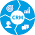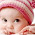## Monday, July 4, 2016

### Monte Carlo Integration in Scala

This post introduces an overlooked numerical integration method leveraging the ubiquituous Monte Carlo simulation.
Not every function has a closed form for computing a definite integral known as symbolic integration. There are many numerical integration method for continuous functions such as Simpson's formula, Newton-Cotes quadrature rule and Gaussian quadrature. These methods are deterministic by nature.
The Monte-Carlo numerical integration is a stochastic method that relies on randomly generated values to estimate the area under a curve, surface or any multidimensional space. The illustration of the Monte Carlo integration method uses a pragmatic uniform random distribution of data points for single variable function.

Basic concept
Let's consider the single variable function illustrated in the following line plot.
$f(x)=\frac{1}{x}-0.5$
The objective is to compute the integral of the function over the interval [1, 3]. The Monte Carlo numerical integration is defined by three steps:
• Define the outer area of the function defined by the minimum and maximum values of x and y, in this case x [1, 3] and y [0.5, -0.12]
• Generate random data points between over the outer area
• Compute the ratio of the number of random data points within the function area over the total number of data points
Let's define a generic class, MonteCarloIntegrator to encapsulate these 3 steps:
The class has two arguments (line 1)
• The function f to sum
• The number of random data points used in the methodology

  1 2 3 4 5 6 7 8 9 10 11 12 13 14 15 16 17 18 19 20 21 22 23 24 25 26 27 28 class MonteCarloIntegrator(f: Double => Double, numPoints: Int) { def integrate(from: Double, to: Double): Double = { val (min, max) = getBounds(from, to) val width = to - from val height = if (min >= 0.0) max else max - min val outerArea = width * height val randomx = new Random(System.currentTimeMillis) val randomy = new Random(System.currentTimeMillis + 42L) def randomSquare(randomx: Random, randomy: Random): Double = { val numInsideArea = Range(0, numPoints)./:(0)( (s, n) => { val ptx = randomx.nextDouble * width + from val pty = randomy.nextDouble * height randomx.setSeed(randomy.nextLong) randomy.setSeed(randomx.nextLong) s + (if (pty > 0.0 && pty < f(ptx)) 1 else if (pty < 0.0 && pty > f(ptx)) -1 else 0) } ) numInsideArea.toDouble * outerArea / numPoints } randomSquare(randomx, randomy) } } 

The method integrate implements the sum of the function over the interval [from, to] (line 3). The first step is to extract the bounds getBounds of the outer area (line 4) which size is computed on line 7. Each coordinate is assigned a random generator randomx and randomy (lines 8 & 9).
The nested method randomSquare records the number of data points, numInsideArea that falls into the area delimited by the function (line 13 - 21). The sum is computed as the relative number of data points inside the area delimited by the function (line 24).

The method getBounds is described in the following code snippet. It is a simple, although not particularly efficient approach to extract the boundary of the integration. It breaks down the interval into of steps (lines 2 & 3) and collects the minimum and maximum values of the function (lines 7 - 12).

  1 2 3 4 5 6 7 8 9 10 11 12 13 14 15 16 17 18 19 20 21 22 23 24 def getBounds(from: Double, to: Double): (Double, Double) = { val numSteps = Math.sqrt(numPoints).floor.toInt val stepSize = (to - from) / numSteps (0 to numSteps)./:((Double.MaxValue, -Double.MaxValue))( (minMax, n) => { val y = f(n * stepSize + from) updateBounds(y, minMax) match { case 0x01 => (y, minMax._2) case 0x02 => (minMax._1, y) case 0x03 => (y, y) case _ => minMax } } ) } def updateBounds(y: Double, minMax: (Double,Double)): Int = { var flag = 0x00 if (y < minMax._1) flag += 0x01 if (y > minMax._2) flag += 0x02 flag } 

Precision, precision
You may wonder about the accuracy of the Monte Carlo method and how many randomly generated data points are needed for a decent accuracy. Let's consider the same function $f(x)=\frac{1}{x}-0.5$ and its indefinite integral, that is used to generated the expected sum for the function f $\int f(x)dx=log(x)-\frac{1}{2x}+C$ The simple test consists of computing the error between the value produced by the definite integral and the sum from the Monte Carlo method as implemented in the following code snippet.

 1 2 3 4 5 6 7 8 val fct =(x: Double) => 2 * x - 1.0 val integral = (x: Double, c: Double) => x*x - x + c) final val numPoints=10000 val integrator = new MonteCarloIntegrator(fct, numPoints) val predicted = monteCarloIntegrator.integrate(1.0, 2.0) val expected = integral(2.0, 0.0) - integral(1.0, 0.0) 

We run the test 100 times for number of random data points varying between 100 and 50,000.

Monte Carlo is reasonably accurate even with a small number of data points. In this case, the small increase in accuracy does not justify the need to randomize a significant number of data points beyond 1000 data points.

A smarter approach would be to rely on a exit condition to complete the summation in the shortest time possible without having to estimate the optimum number of random data points. A new batch of numPoints rand data points is added at each iteration. One simple convergence criteria compares the difference of the sum between two consecutive iterations:
  sum(existing data points + new batch data points) - sum(existing data points) < eps

  1 2 3 4 5 6 7 8 9 10 11 12 13 14 def randomSquare(randomx: Random, randomy: Random): Double = { var oldValue = 0.0 Range(0, numPoints).takeWhile(_ => { val numInsideArea = .... // ... s + .... }) val newValue = numInsideArea.toDouble * outerArea / numPoints val diff = Math.abs(newValue - oldValue) oldValue = newValue diff < eps }) oldValue } 

At each iteration, a new batch of numPoints data points is randomly generated to enhance the accuracy of the summation. The exit strategy is implemented through the higher order Scala collection method takeWhile (lines 3 & 11).

Note: This implementation of the Monte Carlo integration is simple enough to illustrate the concept of stochastic methods applied to calculus. The recursive stratified sampling has been shown to be more accurate for computing definite integral of function with significant inflection points (extreme second order derivative).

References
Monte Carlo Integration Dartmouth College - C. Robert, G. Casella
The Clever Machine: Monte Carlo ApproximationsDustin Stansbury
Programming in Scala - 3rd edition M. Odersky, L. Spoon, B. Venners

#### 4 comments:

1.Very interesting integration of monte carlo in scala. Something that students from http://www.trustessays.com/ will find very useful to use and integrate.

2.I'm back to this nice article, Thanks for sharing and keep sharing.
erp in chennai

3.Your website content nice nice and interesting to observe.
office 2013 professional plus key deutsch

4.Your website content nice nice and interesting to observe.
seo lüdenscheid